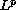Electron. J. Diff. Eqns., Vol. 2002(2002), No. 61, pp. 1-10.

### Existence of solutions for discontinuous functional equations and elliptic boundary-value problems Siegfried Carl & Seppo Heikkila

Abstract:
We prove existence results for discontinuous functional equations in general-spaces and apply these results to the solvability of implicit and explicit elliptic boundary-value problems involving discontinuous nonlinearities. The main tool in the proof is a fixed point result in lattice-ordered Banach spaces proved by the second author.

Submitted January 15, 2002. Published June 25, 2002.
Math Subject Classifications: 35J65, 35R05, 35R10.
Key Words: functional equations, elliptic boundary-value problems, discontinuous nonlinearities.

Show me the PDF file (227K), TEX file, and other files for this article.

Siegfried Carl
Florida Institute of Technology, Department of Mathematical Sciences,
150 West University Boulevard, Melbourne, FL 32901, USA,
e-mail: scarl@fit.edu